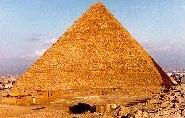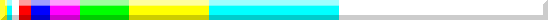GOLDEN SECTION, GROWTH AND FIBONACCI SERIES

Ø (fi), was described by Johannes Kepler as one of the "two great treasures of geometry" (the other was the Pythagorean theorem).
The golden rectangle, in size  Ø e 1, it can be easily built with ruler and compass according to the technique shown in the image below. (click on the image to download the dwg)Starting from the golden rectangle, the golden spiral.It seems that these relationships were known since the time of the Egyptians, as they are found as particular harmonic relationships in the study of the dimensions of the pyramid of Cheops. Similar proportions are also found on the Parthenon in Athens.If you want to build a sequence of relationships in which the golden relationship between the new quantities is constantly preserved, you can proceed as follows:

(1-x):x=x:1=1:(1+x)=(1+x):(2+x)=(2+x):(3+2x)=(3+2x):(5+3x)=(5+3x):(8+5x)=
(8+5x):(13+8x)=(13+8x):(21+13x) ......

The numerical sequences that we have thus obtained indicate precisely the close correlation with the in the development of Fibonacci sequence. With the increase in values, this comes closer and closer to a geometric progression of reason Ø.